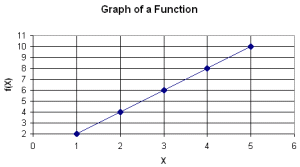Email us to get an instant 20% discount on highly effective K-12 Math & English kwizNET Programs!

#### Online Quiz (WorksheetABCD)

Questions Per Quiz = 2 4 6 8 10

### Grade 5 - Mathematics13.4 Functions and Graphs

 Example: The following graph shows values of the function f(x) for each value of x. What is the function shown in the graph?We find that for 2 on x axis, the corresponding value on y axis is 1 Hence function is f(x) = x/2 Answer: f(x) = x/2 Directions: Find functions for the following graphs. Write at least 5 examples of your own.
 Q 1: The following graph shows values of the function f(x) for each value of x. What is the function shown in the graph?f(x) = x+2f(x) = x*2f(x) = x-2f(x) = x/2 Q 2: The following graph shows values of the function f(x) for each value of x. What is the function shown in the graph?f(x) = x/2f(x) = x*2f(x) = x+2f(x) = x+3 Question 3: This question is available to subscribers only! Question 4: This question is available to subscribers only!